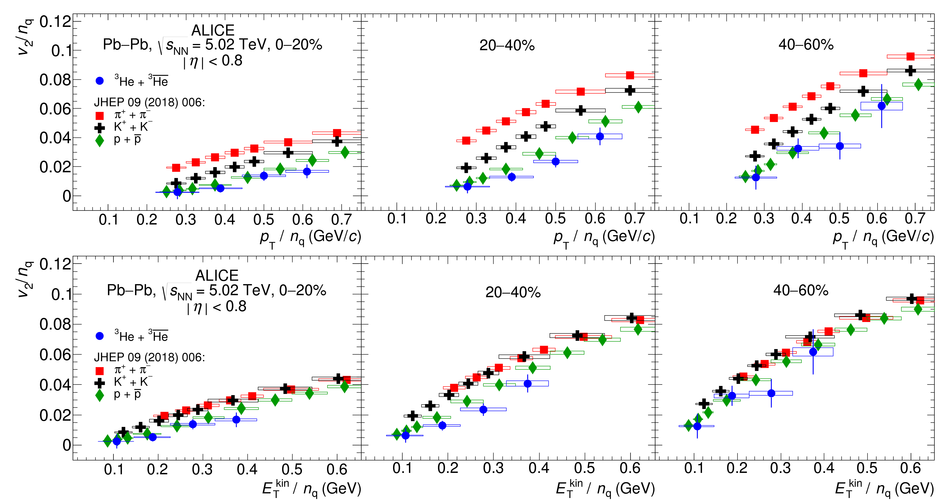# Figure 6

 Comparison between the elliptic flow of pions, kaons, protons, and (anti-)$^{3}$He divided by the number of constituent quarks ($n_{\mathrm{q}}$) as a function of $p_{\mathrm{T}}/n_{\mathrm{q}}$ (upper panels) and transverse kinetic energy per constituent quark $E^{\mathrm{kin}}_{\mathrm{T}}/n_{\mathrm{q}}$ (lower panels) for the centrality classes 0--20$\%$ (left), 20--40$\%$ (middle) and 40--60$\%$ (right). See text for details. Vertical bars and boxes represent the statistical and systematic uncertainties, respectively.